# Intermediate Geometry : How to find the length of an edge of a cube

## Example Questions

1 3 Next →

### Example Question #21 : How To Find The Length Of An Edge Of A Cube

A geometric cube has a volume of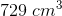. Solve for the length of the edge.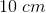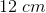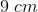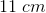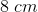Explanation:

The formula for volume of a perfect sphere is represented in this formula, withrepresenting length of sides: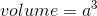Since we know the volume, we can use the formula for volume to determine length of edges: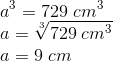1 3 Next →